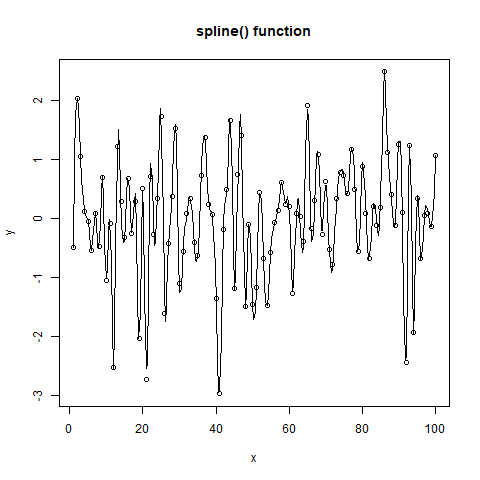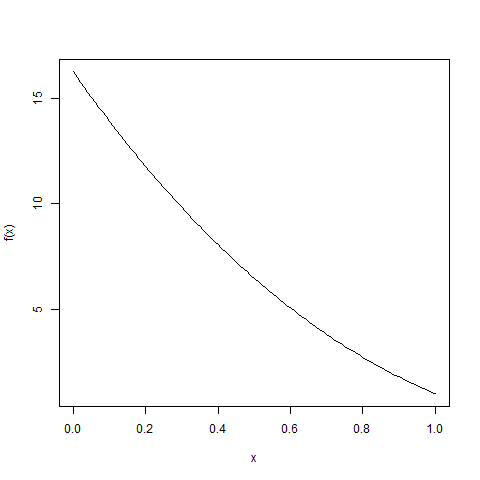Open in App
Not now

# Get a List of points obtained by Interpolation in R Programming – spline() and splinefun() Function

• Last Updated : 03 Jul, 2020

In R programming, `spline()` and `splinefun()` function is used to create a list of points obtained by interpolation. It performs cubic spline interpolation of given data points.

Syntax:
spline(x, y, method)
and
splinefun(x, y, method)

Parameters:
x, y: represents vectors giving the points for interpolation
method: represents the type of spline interpolation to be used

To know about more optional parameters of both the functions, use below command in console:

```help("spline")
```

Example 1:

 `# Coordinates ` `n <- 100 ` `x <- 1:n ` `y <- ``rnorm``(n) ` ` `  `# Output to be present as PNG file ` `png``(file = ``"splineGFG.png"``) ` ` `  `# Spline() function ` `plot``(x, y, main = ``"spline() function"``) ` `lines``(``spline``(x, y)) ` ` `  `# Saving the file ` `dev.off``() `

Output:Example 2:

 `# Coordinates ` `n <- 100 ` `x <- 1:n ` `y <- ``sin``((x-0.5)*``pi``) ` ` `  `# Output to be present as PNG file ` `png``(file = ``"splinefunGFG.png"``) ` ` `  `f <- ``splinefun``(x, y) ` `curve``(``f``(x)) ` ` `  `# Saving the file ` `dev.off``() `

Output:My Personal Notes arrow_drop_up
Related Articles